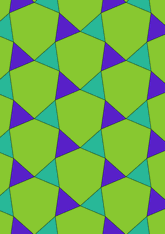# Figure 2.5.7 (d) on page 88data146/F257D

## Geometry

• The symmetry group of the tiling is 3*3 (p31m).
• All the internal angles of the constituent polygons are a multiple of 6°.
• Contains two equilateral triangles.
• Contains one regular three-pointed star polygon with vertex angle of 102°.
• The tiling satisfies the two-colour condition.
• The tiling is edge-to-edge.
• As drawn, contains about 84 polygons.

## References

Publications referenced:
1. Figure 2.5.7 (d), Page 88 of B GrÃ¼nbaum and G C Shephard. Tilings and Patterns, W H Freeman, 1987. ISBN 071671193. [gands] {Most important work in this area.}

v53